﻿ Simulation to Study the Effect of Carrier Concentration on I-V Characteristics of Schottky Diode
Journal of Material Sciences & Engineering
All submissions of the EM system will be redirected to Online Manuscript Submission System. Authors are requested to submit articles directly to Online Manuscript Submission System of respective journal.
• Research Article
• J Material Sci Eng 2015, Vol 5(1): 213
• DOI: 10.4172/2169-0022.1000213

# Simulation to Study the Effect of Carrier Concentration on I-V Characteristics of Schottky Diode

Sharma R*
Department of Applied Sciences, Model Institute of Engineering and Technology, Jammu (J&K), India
*Corresponding Author: Sharma R, Department of Applied Sciences, Model Institute of Engineering and Technology, Jammu (J&K), India, Tel: 9018312123, Email: [email protected]

Received Date: Oct 19, 2015 / Accepted Date: Nov 19, 2015 / Published Date: Nov 29, 2015

### Abstract

The current-voltage characteristics for Au/n-Si Schottky diode are generated by simulation. The simulation performed using Newton-Raphson iteration method yields current-voltage characteristics over wide temperature range. The data is analyzed using TDE-mechanism to study the temperature dependence of barrier height and ideality factor. Results obtained from simulation studies show the barrier height and ideality factor are independent of temperature for pure TE-mechanism. Thus the simulation of I-V characteristics is performed by incorporating ideality factor, obtained on the basis of carrier concentration from 1022-1024 atoms/m3. The result of analysis yield barrier height is still independent of temperature but the ideality factor becomes temperature dependent and this dependence of ideality factor on temperature increases with increase in carrier concentration. Further, the temperature dependence of barrier height and ideality factor is discussed and simulation of current-voltage characteristics is performed.

Keywords: Schottky barrier diode; Carrier concentration; Electrical parameters of schottky diode

#### Introduction

Current transport across Metal-semiconductor (MS) contact is of great interest for device and material scientists. A number of attempts have been made so far to understand this mechanism, but a complete description of the conduction mechanism across the MS interface is still a challenging problem. For an ideal Schottky diode, the current flow is only due to thermionic emission (TE) mechanism with ideality factor equal to unity (η=1). However, due to various factors such as device temperature, dopant concentration, device area, density of interface states, structural properties of interface etc., the current-voltage characteristics of Schottky contact exhibit deviations from TE-mechanism with temperature dependent ideality factor [1-4]. Generally, the ideality factor increases with decrease in temperature, the phenomena is commonly known as “To-effect” and was first proposed by Padovani and Sumner , where as the barrier height for these diodes is found to decrease with fall in operating temperature.

Taking into account the effect of above-mentioned anomalies of the I-V characteristics, different methods have been suggested. Padovani and Stratton proposed, in case of degenerate materials (i.e., ND ≥ 1023 atoms/m3) the above anomalies can be explained on the basis of quantum mechanical tunneling [1,2]. Hackam and Harrop suggested, the ideality factor should be incorporated in saturation current expression . Further, Zs. J. Horvath proposed, any mechanism which enhances electric field at the MS interface is responsible to enhance multistep tunneling at the interface . Accordingly, the temperature dependence of ideality factor as well as barrier height (BH) of Schottky contacts can be explained on the basis of TFE-theory.

Another approach for explaining these anomalies is based on barrier inhomogeneity models. According to these models, barrier height of the contact will be affected by the non-uniformity of the interfacial layer and form a distribution over the contact. As a result, this fluctuation of the barrier height over Schottky contact may lead to non-ideal I-V characteristics [8,9].

Simulation is an emerging trend in the field of research and development and is widely used to study the I-V characteristics of Schottky diode also. S. Chand and J. Kumar  and S. Chand and S. Bala [11,12] have carried out a detailed study on Schottky diode by using simulation. However, all these studies are based on barrier inhomogeneity model (Gaussian). In present paper a different approach has been adopted to explain the temperature dependence of barrier height through simulation of I-V characteristics of Schottky diode.

#### Method Of Simulation

On the basis of Bathe’s thermionic emission theory , the forward I-V characteristics of an ideal Schottky barrier diode can be expressed as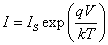(1)

where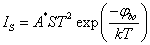(2)

IS is the diode saturation current, A* is the Richardson constant, S is the diode area, φbo is the barrier height, T is the temperature and k is the Boltzman constant. The simulation of I-V characteristics using eq. (1) is performed through computer programming (GW-Basic) using Newton-Raphson iteration method. Parameters used for simulation are: diode area S = 7.87 10-7m2 (corresponding to diameter 1mm), effective Richardson constant A* = 1.12 × 106 Am-2K-2 (for n-type silicon), barrier height φbo = 0.8 eV (Au/n-Si device), and Rs = 10Ω.

#### Results And Discussion

At any temperature, for V >> 3kT/q, eq (1) predicts, the ln(I) vs V plot should be a straight line with unit slope and its intercept at zero bias give IS. Whereas ln(IS/ST2) vs 1/T plot (eq. 2,) should be another straight line with intercept at 1/T = 0 and slope gives the value of A* and φbo respectively. The ln(I)-V plots thus obtained at various temperatures are shown in Figure 1. These curves are linear over several orders of current. I-V data so obtained is analyzed using thermionic emission diffusion (TED) theory to obtain the BH and ideality factor.

Figure 1: Simulated I-V characteristics of Schottky diode with BH = 0.8 eV and Rs = 10 Ω at various temperatures using eq. (1).

Figure 2 shows the BH and ideality factor obtained from simulated data at various temperatures. The barrier height and ideality factor are found to be independent of temperature as expected for the case of pure thermionic emission. But in actual practice the barrier height and ideality factor is generally temperature dependent. Also, on the basis of pure TE – mechanism the simulation is possible upto 125K only. This is because the value of saturation current IS below 125K is extremely low.

Figure 2: Plot showing the variation of ideality factor and barrier height with temperature obtained from I-V data of Figure 1.

However, for diode from heavily doped semiconductor or with imperfection junction, the equation (1) is no longer valid. Podovani and Sumner  reported their non-ideal forward I-V characteristics to fit the expression.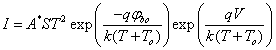where To is called excess temperature. Atalla and Soshea  fit their non-ideal I-V characteristics to the expression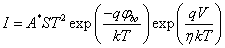(3)

where η is the ideality factor. Padovani and Stratton and later Rhoderick and Williams proposed, if current transport is controlled by the thermionic field emission theory, the relation between current and voltage can be expressed as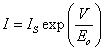(4)

where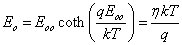and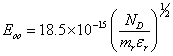The analysis of (4) shows, the ideality factor is temperature dependent through carrier concentration (ND). Thus, I-V data at different temperatures for various values of ND is simulated by incorporating ideality factor in eq. (1).

The I-V data so obtained, at ND = 5 × 1024 atoms/m3 only, is presented in Figure 3. Plot shows the I-V curves are still linear over several orders of current but these are not converging at higher bias voltage in spite of constant value of series resistance (RS). Moreover they are shifting downward and this shift is more prominent at lower temperature. The simulation of I-V data is still possible upto 125K as there is no change in the saturation current. The ideality factor and barrier height obtained from the slope and intercept of ln (I) vs V plot at various temperatures and for different carrier concentrations are presented in Figure 4. Analysis of plot shows, the ideality factor is temperature dependent as observed in most of the cases but the barrier height is still constant (i.e., independent of temperature).

Figure 3: Simulated I-V characteristics of Schottky diode with BH = 0.8 eV, RS= 10 Ω and ND = 5 × 1024 m-3 at various temperatures using eq. (3).

Figure 4: Plot showing the variation of ideality factor and barrier height as a function of temperature, obtained from I-V data shown in Figure 3.

One method to explain the temperature dependence of barrier height and ideality factor is barrier inhomogenities [8,9], which establishes a relation between barrier height and ideality factor also. But this method talks about TED - theory only. Hackam and Harrop  proposed diode equation, for non ideal I-V forward characteristics, as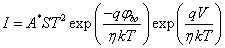(5)

Accordingly, the ideality factor should be included in the expression for saturation current. As the effects that cause deviation from η = 1 at higher bias voltage are present at zero-bias voltage also. Later, Bhuiyan  and more recently Rajinder [16,17], fitted their data by incorporating ideality factor into saturation current.

So, the inclusion of ideality factor in saturation current makes barrier height temperature dependent i.e.,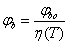(6)

Thus, the simulation of the I-V data of Schottky diode with different carrier concentration has been performed using eq (5) and (6). The I-V data thus obtained is further analyzed on the basis of TED - theory to study the effect of temperature as well as carrier concentration barrier height and ideality factor.

Figure 5a shows I-V curves from 50-300K at ND = 5 × 1024 atoms /m3 only and Figure 5b shows I-V curves at 300, 200 and 125K for various values of ND. A look at plot Figure 5a shows, the I-V characteristics are linear over several orders of current. These curves become more and more linear and linearity of curves shifts towards higher bias voltage with decrease in temperature. This is because of excess current at low temperature due to low barrier height. Also Figure 5b shows there is increase in current with increase in carrier concentration and variation becomes more prominent with decrease in temperature.

Figure 5: (a) Simulated I-V characteristics of Schottky diode with BH = 0.8 eV, Rs = 10 Ω and ND = 5×1024 m-3 at various temperatures using eq. (4) (b) I-V characteristics at different temperatures and various values of ND.

Figure 5a shows I-V curves from 50-300K at ND = 5 × 1024 atoms /m3 only and Figure 5b shows I-V curves at 300, 200 and 125K for various values of ND. A look at plot Figure 5a shows, the I-V characteristics are linear over several orders of current. These curves become more and more linear and linearity of curves shifts towards higher bias voltage with decrease in temperature. This is because of excess current at low temperature due to low barrier height. Also Figure 5b shows there is increase in current with increase in carrier concentration and variation becomes more prominent with decrease in temperature.

The I-V data so obtained, for various values of ND, is analyzed on the basis of thermionic emission theory. The barrier height and ideality factor as a function of temperature are shown in Figure 6. Plot shows the barrier height decreases and ideality factor increases with decrease in temperature in the same manner as most of the practical Schottky diodes exhibit and these changes become more prominent with increase in the donor concentration (ND) and decrease in temperature.

Figure 6: Variation of ideality factor and barrier height as a function of temperature at different values of ND.

The value of IS at each temperature, obtained from Figure 5a, is used to plot ln(Is/T2) vs 1/T as shown in Figure 7, the usual Richardson plot (curve-a). For an ideal diode this should be a straight line with intercept and slope yielding the value of A* and BH respectively. But the extracted data exhibit linearity upto 200K only with A* = 2.15 × 103 Am-2K-2 and BH = 0.60 eV. Clearly the values of A* and BH, so obtained are very less as compared to the values used for simulation. This discrepancy is because of extra current at low temperature. Further, the data is analyzed by using modified Richardson plot i.e., ln(Is/T2) vs 1/ηT. Such a plot is shown in Figure 7 (curve-b), exhibit linearity over entire temperature range yielding the value of A* = 1.13 × 106 Am-2K-2 and BH = 0.8 eV and these values are in good agreement with those used for simulation.

#### Conclusion

The I-V characteristics of Schottky diodes are simulated over wide temperature range. The I-V data for Au/n-Si Schottky diode is generated with and without ideality factor as well as by incorporating ideality factor in saturation current equation. The data so obtained fitted into the pure TED-equation to see the temperature dependence of ideality factor and barrier height. For I-V characteristics with η=1, the barrier height and ideality factor are independent of temperature. Whereas incorporation of ideality factor in saturation current makes barrier height temperature dependent. It is also observed that the temperature dependence of barrier height becomes stronger with increase in carrier concentration. Finally it is concluded that the temperature dependence of barrier height is due to the factors which are responsible for the deviation of I-V characteristics of Schottky diodes at higher bias voltage.

#### References

Citation: Sharma R (2015) Simulation to Study the Effect of Carrier Concentration on I-V Characteristics of Schottky Diode. J Material Sci Eng 5: 213. Doi: 10.4172/2169-0022.1000213

Copyright: © 2015 Sharma R. This is an open-access article distributed under the terms of the Creative Commons Attribution License, which permits unrestricted use, distribution, and reproduction in any medium, provided the original author and source are credited.

Select your language of interest to view the total content in your interested language

##### Recommended Journals
Viewmore
• ###### 24th International Conference on Advanced Materials & Nanotechnology

September 19-20, 2019 Brussels, Belgium

• ###### World Congress on Carbon and Advanced Energy Materials

September 23-24, 2019 Hong Kong, Thailand

• ###### 21st International Conference on Advanced Materials Science & Nano Technology

September 26-27, 2019 Dubai, UAE

• ###### 5th International Conference on Crystallography & Novel Materials

November 18-19, 2019 Helsinki, Finland

• ###### 10th International Conference on Biopolymers and Polymer Sciences

November 18-19, 2019 Helsinki, Finland

Viewmore
###### Article Usage
• Total views: 9261
• [From(publication date): 2-2016 - Aug 23, 2019]
• Breakdown by view type
• HTML page views: 9112
• PDF downloads: 149

## Post your commentCan't read the image? click here to refresh
###### Peer Reviewed Journals

Make the best use of Scientific Research and information from our 700 + peer reviewed, Open Access Journals

International Conferences 2019-20

Meet Inspiring Speakers and Experts at our 3000+ Global Annual Meetings

Top